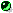Electron Transport Coefficients of Magnetized Stellar Plasmas Electrical and Thermal Conductivities and Thermopower of fully ionized plasma in arbitrary magnetic field Department of Theoretical Astrophysics [Conductivity Main page] [Neutron Star Group]
[click here to go straight to the Fortran programs]

Description

Here we present Fortran programs for calculation of all components of kinetic tensors in fully ionized electron-ion plasmas at arbitrary magnetic field. There are two versions of the code: one of them (the "short" one, ~ 20 K) is designed for conditions at which the electrons are very strongly degenerate - that is, it sets all scattering electrons on the Fermi surface while calculating the electrical conductivity, whereas the thermal conductivity is obtained using the Wiedemann-Franz law. This version of the code is pretty fast and relatively simple. The second version (the "long" one, ~ 40 K) employs a thermal averaging with the Fermi distribution function; this version can be used when electrons are partially degenerate; it provides, along with the electrical and thermal conductivities, also thermopower (thermoelectric coefficient).

Both versions of the program take into account collisions of the electrons with ions and (in solid phase) charged impurities. Quantum effects on ionic motion are taken into account in the solid phase only. Influence of the latter effects on the conductivities has been studied accurately in the nonmagnetic case only. For this reason, we do not take into account the difference between effective relaxation times for electrical and thermal conductivities if magnetic field strength B is non-zero. Therefore, whenever these effects are important (i.e., at temperature T much smaller than the ion plasma temperature), the conductivity with magnetic field is calculated less accurately.

Similarly, electron-electron collisions are taken into account only for B=0. Thus, you should set B=0 (exactly) if you are interested in most accurate nonmagnetic conductivity values.

Each of the versions uses the ion charge number Z, mass number A, impurity parameter Zimp, B, T, and density \rho as input parameters. Output of the "short" version are the longitudinal, transverse, and off-diagonal (Hall) components of electrical and thermal conductivity tensors. In the "long" version, the components of thermoelectric tensor are produced in addition.

If compiled "as is," each of the programs displays the output on terminal. However, the first (MAIN) routine can be easily modified to yield another output. Alternatively, the MAIN routine can be commented-out for usage of the principal subroutine CONDEGEN (in the "short" version) or CONDUCT (in the "long" one) with another package.

For theoretical background, see the main page. Some explanations, meant to facilitate adaptation of different subroutines to your particular needs, are given in comments to the subroutines."short" version"long" version